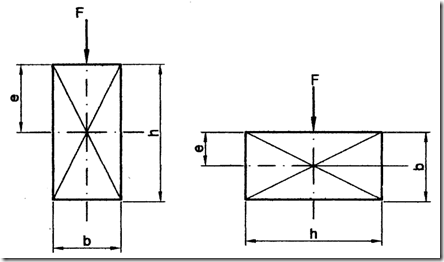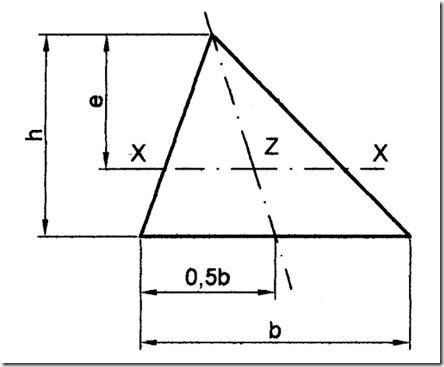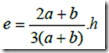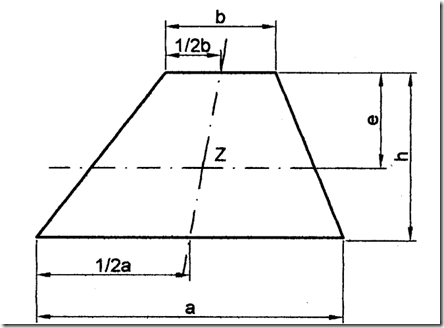Home > English > Regular Sector Weight Point

# Regular Sector Weight Point

The emphasis of a flat field beratuaran or can be seen in the following figure:
1. Cross-section ampat terms.
For a rectangular cross-section has a length and width h mm b mm, where the emphasis is the intersection point of the diagonal or e = ½ h mm for the vertical cross section and size e = ½ b mm for horizontal cross-section.
See the following figure.Figure 4.4 Ttitk heavy rectangular cross-section

2. Cross-section square
Location of the center of gravity for the fields that form a square that has the size of a mm, the size e = ½ a (mm), see the following pictureFigure 4.5 The weight of a square cross-section

3. Cross-section circle
Location of the center of gravity for the field that has the shape of a circle with a diameter d mm is at its center point, ie e = ½ d (mm) or see the following picture.Figure 4.6 The emphasis of circular cross section

4. The triangular cross-section
Location of the center of gravity for the fields that form a triangle which has the size of the base b (mm) and height h (mm), then the position of the emphasis is on the point Z and sizes: e = 2/3 h (mm), see the following pictureFigure 4.7 The emphasis for the triangular cross section

5. Sectional trapezium
Location of the center of gravity for the fields that form the trapezium which has the size of the pedestal a (mm), the upper side b (mm) and height h (mm), then the position of the emphasis is on the point Z see the picture and size: e is calculated by the following equation:Figure 4.8 The emphasis for sectional trapezium